Next: Bayesian statistics: learning by Up: Conditional probability and Bayes' Previous: Bayes' theorem   Contents

## Conventional use of Bayes' theorem

Bayes' theorem follows directly from the rules of probability, and it can be used in any kind of approach. Let us take an example:
Problem 1:
A particle detector has a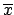identification efficiency of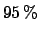, and a probability of identifying a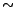as aof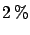. If a particle is identified as a, then a trigger is fired. Knowing that the particle beam is a mixture ofand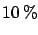, what is the probability that a trigger is really fired by a? What is the signal-to-noise (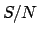) ratio?
Solution:
The two hypotheses (causes) which could condition the event (effect)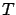(=trigger fired'') are'' and''. They are incompatible (clearly) and exhaustive (90% + 10% = 100%). Then: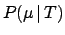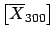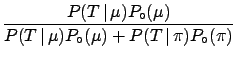(3.16) (3.17)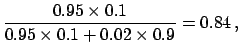and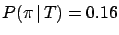.

The S/N ratio is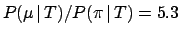. It is interesting to rewrite the general expression of the S/N ratio if the effect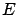is observed as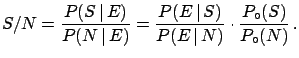(3.18)

This formula explicitly shows that when there are noisy conditions,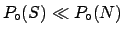, the experiment must be very selective,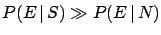, in order to have a decentratio.
(How doeschange if the particle has to be identified by two independent detectors in order to give the trigger? Try it yourself, the answer is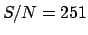.)
Problem 2:
Three boxes contain two rings each, but in one of them they are both gold, in the second both silver, and in the third one of each type. You have the choice of randomly extracting a ring from one of the boxes, the content of which is unknown to you. You look at the selected ring, and you then have the possibility of extracting a second ring, again from any of the three boxes. Let us assume the first ring you extract is a gold one. Is it then preferable to extract the second one from the same or from a different box?
Solution:
Choosing the same box you have a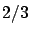probability of getting a second gold ring. (Try to apply the theorem, or help yourself with intuition; the solution is given in Section.)
The difference between the two problems, from the conventional statistics point of view, is that the first is only meaningful in the frequentistic approach, the second only in the combinatorial one. They are, however, both acceptable from the Bayesian point of view. This is simply because in this framework there is no restriction on the definition of probability. In many and important cases of life and science, neither of the two conventional definitions are applicable.Next: Bayesian statistics: learning by Up: Conditional probability and Bayes' Previous: Bayes' theorem   Contents
Giulio D'Agostini 2003-05-15